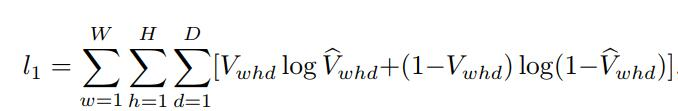# Calc Loss Resulting in Out of Memory

The origin Loss Function is ,and W = 192, H = 192, D = 200
Thus I worte a Loss Class,

``````class CrossEntropyLoass(nn.Module):
def __init__(self):
super(CrossEntropyLoass, self).__init__()
return

'''test is V, output is V^'''
def forward(self, output, test):
start_time = time.time()
count, d, w, h = test.shape
losses = 0
for i in range(w):
for j in range(h):
for k in range(d):

losses += torch.mul(test[k][i][j],  torch.log(output[k][i][j])) + torch.mul((1-test[k][i][j]) ,  torch.log(1 - output[k][i][j]))

cost_time = time.time() - start_time
print('Costed time {:.0f}m {:.0f}s'.format(cost_time // 60, cost_time % 60))
return losses
``````

it’s called by

``````loss = criterion(output, test_vol)
``````

the shape of output and test_vol are `torch.Size([1, 200, 192, 192])`.
But it seems take up too much memory, I haven’t finished calc one loss, it shut down with errors out of memory.

``````THCudaCheck FAIL file=c:\users\administrator\downloads\new-builder\win-wheel\pytorch\aten\src\thc\generic/THCStorage.cu line=58 error=2 : out of memory
Traceback (most recent call last):
File "E:/2018/formal/pytorch/human_pose/train.py", line 184, in <module>
main()
File "E:/2018/formal/pytorch/human_pose/train.py", line 172, in main
loss = criterion(output, vol)
File "C:\ProgramData\Anaconda3\lib\site-packages\torch\nn\modules\module.py", line 491, in __call__
result = self.forward(*input, **kwargs)
File "E:/2018/formal/pytorch/human_pose/train.py", line 89, in forward
losses += torch.mul(test[k][i][j],  torch.log(output[k][i][j])) + torch.mul((1-test[k][i][j]) ,  torch.log(1 - output[k][i][j]))
File "C:\ProgramData\Anaconda3\lib\site-packages\torch\tensor.py", line 317, in __rsub__
return -self + other